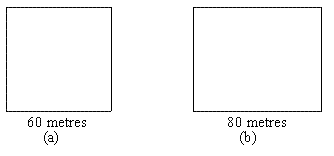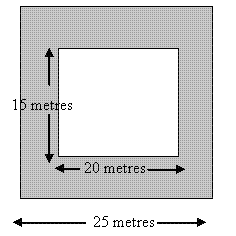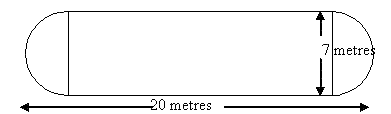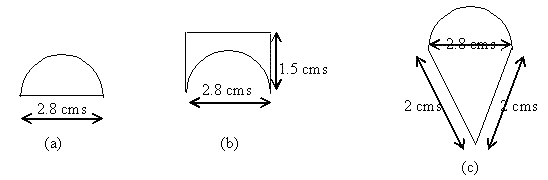# NCERT solutions for class 8 maths chapter 11

In this page we have NCERT solutions for class 8 maths chapter 11 for Exercise 11.1 on Mensuration . This exercise has question on area of square,rectange, triangle,parallelogram and circle. Hope you like them and do not forget to like , social share and comment at the end of the page.

## NCERT solutions for class 8 maths chapter 11 Exercise 11.1

Question 1
A square and a rectangular field with measurements as given in the figure have the same perimeter. Which field has a larger area?Answer: Perimeter of Square   is given by
= 4 x side = 4 x 60 = 240 m
Area of Square = Side² = 60² = 3600 m2
Now Perimeter of Rectangle is given by
Now both the square and rectangle has the same perimeter
So
Breadth = 120-80 = 40 m
Area of rectangle = length x breadth = 80 x 40 = 3200 m2
Now it is clear that the area of the square field is greater than the area of the rectangular field.

Question 2
Mrs. Kaushik has a square plot with the measurement as shown in the figure. She wants to construct a house in the middle of the plot. A garden is developed around the house. Find the total cost of developing a garden around the house at the rate of Rs 55 per m².Answer: Area of the square plot = Side² = 25² = 625 m2
Area of the house construction part = length x breadth
= 20 x 15 = 300 m2
So, area of the garden = Area of square plot – area of house in construction=625-300=325 m2
Cost of developing the garden = Area  of Garden x Rate
= 300 x 55 = 16500 rupees

Question 3
The shape of a garden is rectangular in the middle and semicircular at the ends as shown in the diagram. Find the area and the perimeter of this garden [Length of rectangle is 20 – (3.5 + 3.5) m].= 20 x 7 = 140 sq m
Area of Semicircular portions: = π x r2
Here π=22/7   and radius =7/2=3.5 m
So area of two Semicircular portions: =Area of circle= ? x r2
= (22/7) × 3.5 ×3.5= 37.5
So Total area of the garden=
Area of rectangular portion + Area of circle
=140+37.5=177.5 m2
Now the perimeter of shape= Perimeter of semicircular portion + Length+ Perimeter of semicircular portion + length
=Perimeter of Circle + 2 Length
= 2 π r + 40
=22+40=62 m

Question 4
A flooring tile has the shape of a parallelogram whose base is 24 cm and the corresponding height is 10 cm. How many such tiles are required to cover a floor of area 1080 m2? (If required you can split the tiles in whatever way you want to fill up the corners).
Answer: Area of the Parallelogram = base x height
= 24 x 10 = 240 cm2
So required Number of tiles = Area of the Floor/Area of the Tiles
= 1080 X100 X100/240  =45000
(area of floor is converted into square cm)

Question 5
An ant is moving around a few food pieces of different shapes scattered on the floor. For which food-piece would the ant have to take a longer round? Remember, circumference of a circle can be obtained by using the expression c = 2πr, where r is the radius of the circle.1.  In the first case, Perimeter of the shape = Perimeter of Semicircular part + Diameter of the part
= π r + 2r
=(22/7) × (1.4)  + 2.8
=7.2 cm
2. In the second case, Perimeter of the shape = Perimeter of Semicircular part + 2Length + Breath
= πr +2 L + B
=4.4 + 3 +2.8
=10.2 cm
3. In the third case, Perimeter of the shape = Perimeter of Semicircular part + 2(slant height)
=4.4 + 4=8.4 cm

So, the food shape in (a) requires the ant to cover the least distance.

## Summary

1. NCERT solutions for class 8 maths chapter 11 Exercise 11.1 has been prepared by Expert with utmost care. If you find any mistake.Please do provide feedback on mail. You can download the solutions as PDF in the below Link also
2. This chapter 11 has total 4 Exercise 11.1 ,11.2 ,11.3 and 11.4. This is the First exercise in the chapter.You can explore previous exercise of this chapter by clicking the link below